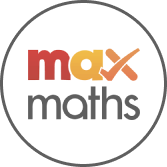# Multiplying by 5 and 10

Lesson
Maths
Year 2Max Maths, Year 2, Learn together, Multiplying by 5 and 10 (1)
Resource 1/10Max Maths
Max Maths is a specialist primary school maths provider that uses the Singapore Mastery Approach to follow the Cambridge Primary maths curriculum framework.